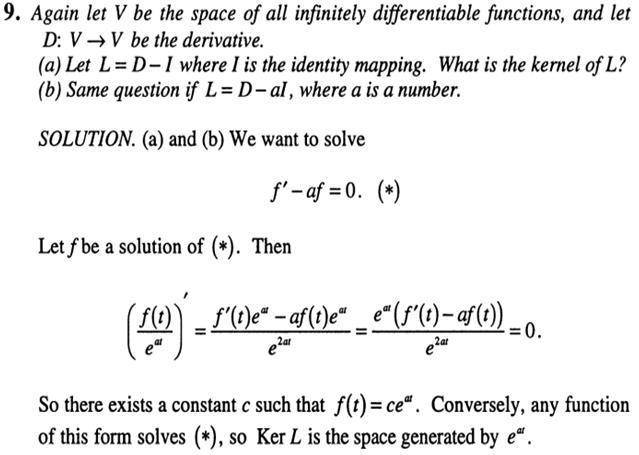# Vector spaces problem -linear algebra

manuel325

## Homework Statement

Hi guys , I have this problem ,well actually I don't understand the solution they provide , Here's the problem statement and the solution .May someone please explain the solution to me?? Thanks so much, Sorry for my bad english

## Homework Equations

1.I understand that f'-af=0 and the kernel is the space of the solutions that satisfy that equation but I don't get what they do after that...why do they divide f(t) by e^(at)?
2. why do they conclude that exists a constant c such that f(t)=ce^at??

## Answers and Replies

Homework Helper
The problem is this: we have the vector space of all infinitely differentiable functions, D is the differentiation operator.
a) Find the kernel of the linear operator D- I.
b) Find the kernel of the linear operator D- aI.

It is simplest to solve (b) first, then take a= 1 to solve (a).
If f is any function in the kernel of D- aI then, by definition of "kernel" we must have f'- af= 0.
That is the same as df/dx= af which is a separable equation: $$df/f= adx$$. Integrating both sides, ln(f)= ax+ d where c is the constant of integration. Taking the exponential of both sides $f(x)= e^{ax+ c}= Ce^{ax}$ where $C= e^c$.

That is how I would have solved the problem. I suspect that your text, knowing that f must be an exponential, started from that:
$$\left(\frac{f(x)}{e^{ax}}\right)'= \frac{f'(x)e^{ax}- f(x)ae^{ax}}{e^{2ax}}= \frac{(f'(x)- af(x))e^{ax}}{e^{2ax}}$$
by the product rule.

And, because $f'(x)- af(x)= 0$, the right side is 0, the derivative of $f(x)/e^{ax}$ is 0 so that $f(x)/e^{ax}$ is a constant: $f(x)/e^{ax}= C$ so $f(x)= Ce^{ax}$.

(And your English is excellent. Far better than my (put language of your choice here).

manuel325
Thank you so much HallsofIvy , I understood all your explanation . It's cool to know that there's still good people who like to help others .Greetings from Chile